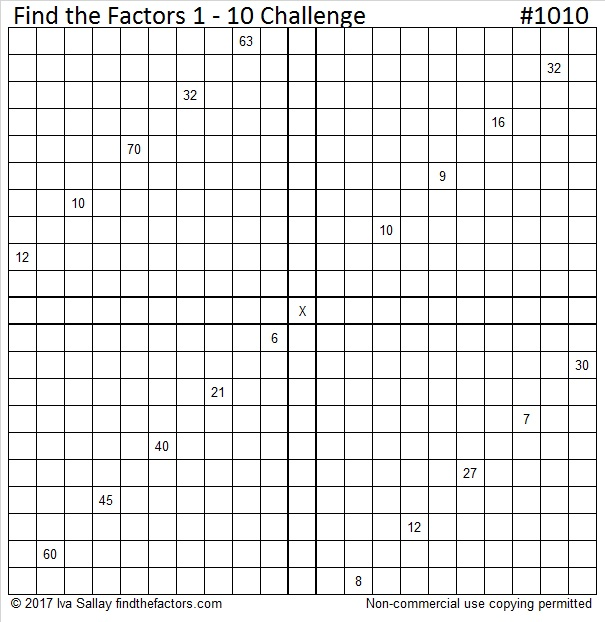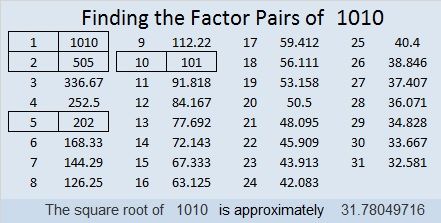# 1019 An Easier Find the Factors Challenge Puzzle

I’ve recently posted some more challenging puzzles that I’ve named Find the Factors 1 – 10 Challenge, and they definitely are a more challenging puzzle than one of my more traditional level 6 puzzles. As of today, no one has informed me that they have been able to solve either puzzle number 1000 or 1010.

Two years ago I made perhaps my most challenging level 6 puzzle, a 16 × 16 puzzle to commemorate Steve Morris’s birthday. Steve Morris was the very first person to type a comment on my blog, and I have appreciated his encouragement over the years. Steve has solved many kinds of puzzles in his life including some of the toughest I have made, but the puzzle I made for that birthday was no picnic for even him to complete.

This year I’ve made him a challenging puzzle, but it is still a little easier than the other two challenge puzzles I’ve made. If you’ve tried either of those other puzzles without success, still give this one a try. Good luck to you all, and Happy Birthday to Steve Morris! I saved this post number (1019) for you because it uses your birthdate numbers, howbeit out of order.Print the puzzles or type the solution in this excel file: 10-factors-1019-1027

This is my 1019th post. Here are a few facts about the number 1019.

Prime number 1019 is the sum of the 19 prime numbers from 17 to 97.

• 1019 is a prime number.
• Prime factorization: 1019 is prime.
• The exponent of prime number 1019 is 1. Adding 1 to that exponent we get (1 + 1) = 2. Therefore 1019 has exactly 2 factors.
• Factors of 1019: 1, 1019
• Factor pairs: 1019 = 1 × 1019
• 1019 has no square factors that allow its square root to be simplified. √1019 ≈ 31.921779

How do we know that 1019 is a prime number? If 1019 were not a prime number, then it would be divisible by at least one prime number less than or equal to √1019 ≈ 31.9. Since 1019 cannot be divided evenly by 2, 3, 5, 7, 11, 13, 17, 19, 23, 29 or 31, we know that 1019 is a prime number.# 1010 Find the Factors Challenge

Level 6 puzzles are difficult. At least they are until you’ve done a few, then they become much less difficult to solve.

This Find the Factors 1 – 10 Challenge is much more difficult. I published the first of these puzzles (#1000) last week, but I won’t necessarily make one every week. If you’ve done a few level 6 puzzles, see how you do with a Challenge Puzzle.Print the puzzles or type the solution in this excel file: 10-factors-1002-1011

Here’s a little about the number 1010:

1010 is the sum of two squares two different ways:
29² + 13² = 1010
31² + 7² = 1010

That means that 1010 is the hypotenuse of some Pythagorean triples:
200-990-1010 which is 10 times (20-99-101)
434-912-1010 calculated from 2(31)(7), 31² – 7², 31² + 7²
606-808-1010 which is (3-4-5) times 202
672-754-1010 calculated from 29² – 13², 2(29)(13), 29² + 13²

1010 is a fun-looking number in base 10.
It is also palindrome 262 in BASE 21 because 2(21²) + 6(21) + 2(1) = 1010

• 1010 is a composite number.
• Prime factorization: 1010 = 2 × 5 × 101
• The exponents in the prime factorization are 1, 1, and 1. Adding one to each and multiplying we get (1 + 1)(1 + 1)(1 + 1) = 2 × 2 × 2 = 8. Therefore 1010 has exactly 8 factors.
• Factors of 1010: 1, 2, 5, 10, 101, 202, 505, 1010
• Factor pairs: 1010 = 1 × 1010, 2 × 505, 5 × 202, or 10 × 101
• 1010 has no square factors that allow its square root to be simplified. √1010 ≈ 31.780497# 1000 Find the Factors Challenge

Dan Bach asked me if I had anything special planned for my 1000 post. I had a few things planned, but this Find the Factors Challenge exists because of his question and our twitter conversation. I like this puzzle better than my previous plans. It is only fitting that I dedicate this puzzle to Dan Bach.

There are four places outlined in bold that need you to place the numbers 1 – 10 so that each section of the puzzle will work as a multiplication table. Each section of the puzzle is dependent on the other sections. None of the sections could be a puzzle independent of the others. You will use similar logic to solve this puzzle as you would a regular Find the Factors puzzle. It will not be easy to solve at all, but give it a try. There is only one solution.Print the puzzles or type the solution in this updated excel file: 12 factors 993-1001

1000 is a perfect cube as well as the product of perfect cubes.

30² + 10² = 1000
26² + 18² = 1000

1000 is the hypotenuse of three Pythagorean triples:
600² + 800² = 1000²
352² + 936² = 1000²
280² + 960² = 1000²

1000 looks interesting in a few bases:
2626 in BASE 7
1331 in BASE 9
1A1 in BASE 27 (A is 10 base 10)

• 1000 is a composite number.
• Prime factorization: 1000 = 2 × 2 × 2 × 5 × 5 × 5, which can be written 1000 = 2³ × 5³
• The exponents in the prime factorization are 3 and 3. Adding one to each and multiplying we get (3 + 1)(3 + 1) = 4 × 4 = 16. Therefore 1000 has exactly 16 factors.
• Factors of 1000: 1, 2, 4, 5, 8, 10, 20, 25, 40, 50, 100, 125, 200, 250, 500, 1000
• Factor pairs: 1000 = 1 × 1000, 2 × 500, 4 × 250, 5 × 200, 8 × 125, 10 × 100, 20 × 50, or 25 × 40
• Taking the factor pair with the largest square number factor, we get √1000 = (√100)(√10) = 10√10 ≈ 31.622777